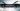Shortcut of Number systemShortcut 77:
Divisibility test
Divisibleby                                    Rule

2                            Last digit of a number should be 0,2,4,6, or 8

3                            Sum of the digit should be divisible by 3

4                            Last two digits of a number should be divisible by 4

5                            Last digit of a number should be 0 or 5

6                             Number should be divisible by 2 and 3

8                             Last three digit of a number shoul be divisible by 8

9                             Sum of the digit should be divisible by 9

10                           Last three digit of a number shoul be 0

11                           Difference between sum of digits in even places shoud be 0 or 11
Question:
Which of the following numbers is divisible by both 5 and 9?
1115, 11115, 111115, 1111115
11115 – Its is divisible by 5 and 9

Shortcut 78:
Finding unit digit
Base Value                                            Power Value

Unit Place                                              Unit Place

0                                                               0

1                                                               1

5                                                               5

6                                                               6
Question:
Find the units place in the result of the expression:
240234 + 345344 x 1011009 – 36211
Unit’s place of 240234 = 0
Unit’s place of 345344 = 5
Unit’s place of 1011009 = 1
Unit’s place of 36211 = 6
Units place of the expression
= 0 + 5 x 1 – 6 = 9
(When subtracting 5 from 6 in unit’s place we will borrow 1 for 5, 15 -6 = 9)

Shortcut 79:
Finding unit digit
Odd           Even

4                       4                6

9                       9                 1
Question:
Find the unit’s digit of 3449 and 4934.
3449 = 4 power odd number – 4 in unit’s place
So, unit digit of 3449 = 4
4934 = 9 power even number – 1 in unit’s place
So, unit’s digit of  4934 = 1

Shortcut 80:
Finding unit digitQuestion:
Find the unit digit of the expression
1211 + 1312 + 1718 + 1817
Unit’s place of 1211 = 8
Unit’s place of 1312 = 1
Unit’s place of 1718 = 9
Unit’s place of 1817 = 8
Sum of unit’s places = 8 + 1 + 9 + 8 = 26
Unit’s digit = 6

Shortcut 81:
Finding remainder
R[(a*b*c)/p]=R(a/p)*R(b/p)*R(c/p)
Question:
Find the remainder when 212 x 313 x 414 is divided by 5.
Using the above concept we can find the remainder for each term and then we can multiply.
R(212/5) = 2
R(313/5) = 3
R(414/5) = 4
2 x 3 x 4 = 24
The value we got is still greater than 5, so again divide it and find the remainder.
R(24/5) = 4
The remainder of the expression is = 4